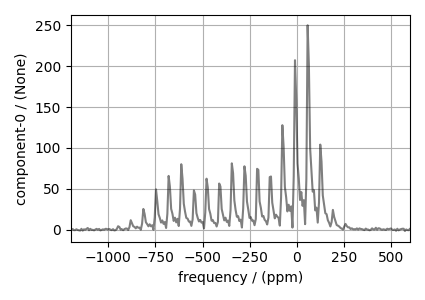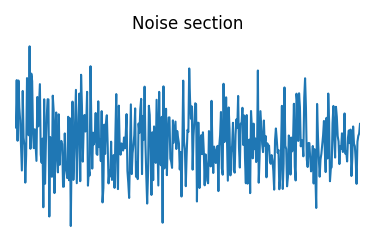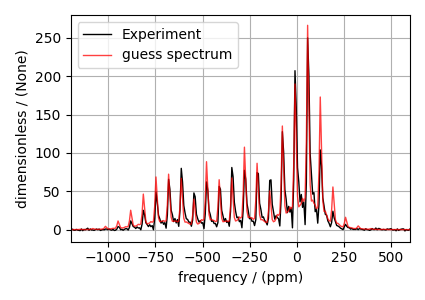# ¹¹⁹Sn MAS NMR of SnO¶

The following is a spinning sideband manifold fitting example for the 119Sn MAS NMR of SnO. The dataset was acquired and shared by Altenhof et al. .

import csdmpy as cp
import matplotlib.pyplot as plt
from lmfit import Minimizer

from mrsimulator import Simulator, SpinSystem, Site, Coupling
from mrsimulator.method.lib import BlochDecaySpectrum
from mrsimulator import signal_processor as sp
from mrsimulator.utils import spectral_fitting as sf
from mrsimulator.utils import get_spectral_dimensions
from mrsimulator.spin_system.tensors import SymmetricTensor


## Import the dataset¶

filename = "https://ssnmr.org/sites/default/files/mrsimulator/119Sn_SnO.csdf"

# standard deviation of noise from the dataset
sigma = 0.6410905

# For spectral fitting, we only focus on the real part of the complex dataset
experiment = experiment.real

# Convert the coordinates along each dimension from Hz to ppm.
_ = [item.to("ppm", "nmr_frequency_ratio") for item in experiment.dimensions]

# plot of the dataset.
plt.figure(figsize=(4.25, 3.0))
ax = plt.subplot(projection="csdm")
ax.plot(experiment, "k", alpha=0.5)
ax.set_xlim(-1200, 600)
plt.grid()
plt.tight_layout()
plt.show()## Create a fitting model¶

Guess model

Create a guess list of spin systems. There are two spin systems present in this example, - 1) an uncoupled $$^{119}\text{Sn}$$ and - 2) a coupled $$^{119}\text{Sn}$$-$$^{117}\text{Sn}$$ spin systems.

sn119 = Site(
isotope="119Sn",
isotropic_chemical_shift=-210,
shielding_symmetric=SymmetricTensor(zeta=700, eta=0.1),
)
sn117 = Site(
isotope="117Sn",
isotropic_chemical_shift=0,
)
j_sn = Coupling(
site_index=[0, 1],
isotropic_j=8150.0,
)

sn117_abundance = 7.68  # in %
spin_systems = [
# uncoupled spin system
SpinSystem(sites=[sn119], abundance=100 - sn117_abundance),
# coupled spin systems
SpinSystem(sites=[sn119, sn117], couplings=[j_sn], abundance=sn117_abundance),
]


Method

# Get the spectral dimension parameters from the experiment.
spectral_dims = get_spectral_dimensions(experiment)

MAS = BlochDecaySpectrum(
channels=["119Sn"],
magnetic_flux_density=9.395,  # in T
rotor_frequency=10000,  # in Hz
spectral_dimensions=spectral_dims,
experiment=experiment,  # add the measurement to the method.
)

# Optimize the script by pre-setting the transition pathways for each spin system from
# the method.
for sys in spin_systems:
sys.transition_pathways = MAS.get_transition_pathways(sys)


Guess Spectrum

# Simulation
# ----------
sim = Simulator(spin_systems=spin_systems, methods=[MAS])
sim.run()

# Post Simulation Processing
# --------------------------
processor = sp.SignalProcessor(
operations=[
sp.IFFT(),
sp.apodization.Exponential(FWHM="1500 Hz"),
sp.FFT(),
sp.Scale(factor=5000),
]
)
processed_dataset = processor.apply_operations(dataset=sim.methods.simulation).real

# Plot of the guess Spectrum
# --------------------------
plt.figure(figsize=(4.25, 3.0))
ax = plt.subplot(projection="csdm")
ax.plot(experiment, "k", linewidth=1, label="Experiment")
ax.plot(processed_dataset, "r", alpha=0.75, linewidth=1, label="guess spectrum")
ax.set_xlim(-1200, 600)
plt.grid()
plt.legend()
plt.tight_layout()
plt.show()## Least-squares minimization with LMFIT¶

Use the make_LMFIT_params() for a quick setup of the fitting parameters.

params = sf.make_LMFIT_params(sim, processor, include={"rotor_frequency"})

# Remove the abundance parameters from params. Since the measurement detects 119Sn, we
# also remove the isotropic chemical shift parameter of 117Sn site from params. The
# 117Sn is the site at index 1 of the spin system at index 1.
params.pop("sys_0_abundance")
params.pop("sys_1_abundance")
params.pop("sys_1_site_1_isotropic_chemical_shift")

# Since the 119Sn site is shared between the two spin systems, we add constraints to the
# 119Sn site parameters from the spin system at index 1 to be the same as 119Sn site
# parameters from the spin system at index 0.
lst = [
"isotropic_chemical_shift",
"shielding_symmetric_zeta",
"shielding_symmetric_eta",
]
for item in lst:
params[f"sys_1_site_0_{item}"].expr = f"sys_0_site_0_{item}"

print(params.pretty_print(columns=["value", "min", "max", "vary", "expr"]))

Name                                      Value      Min      Max     Vary     Expr
SP_0_operation_1_Exponential_FWHM          1500     -inf      inf     True     None
SP_0_operation_3_Scale_factor              5000     -inf      inf     True     None
mth_0_rotor_frequency                     1e+04     9900 1.01e+04     True     None
sys_0_site_0_isotropic_chemical_shift      -210     -inf      inf     True     None
sys_0_site_0_shielding_symmetric_eta        0.1        0        1     True     None
sys_0_site_0_shielding_symmetric_zeta       700     -inf      inf     True     None
sys_1_coupling_0_isotropic_j               8150     -inf      inf     True     None
sys_1_site_0_isotropic_chemical_shift      -210     -inf      inf    False sys_0_site_0_isotropic_chemical_shift
sys_1_site_0_shielding_symmetric_eta        0.1        0        1    False sys_0_site_0_shielding_symmetric_eta
sys_1_site_0_shielding_symmetric_zeta       700     -inf      inf    False sys_0_site_0_shielding_symmetric_zeta
None


Solve the minimizer using LMFIT

minner = Minimizer(sf.LMFIT_min_function, params, fcn_args=(sim, processor, sigma))
result = minner.minimize()
result


## Fit Statistics

 fitting method leastsq # function evals 65 # data points 1000 # variables 7 chi-square 33637.4508 reduced chi-square 33.8745728 Akaike info crit. 3529.64005 Bayesian info crit. 3563.99434

## Variables

name value standard error relative error initial value min max vary expression
sys_0_site_0_isotropic_chemical_shift -207.087935 0.05617007 (0.03%) -210.0 -inf inf True
sys_0_site_0_shielding_symmetric_zeta 646.836110 1.65393575 (0.26%) 700.0 -inf inf True
sys_0_site_0_shielding_symmetric_eta 0.10812413 0.01161821 (10.75%) 0.1 0.00000000 1.00000000 True
sys_1_site_0_isotropic_chemical_shift -207.087935 0.05617007 (0.03%) -210.0 -inf inf False sys_0_site_0_isotropic_chemical_shift
sys_1_site_0_shielding_symmetric_zeta 646.836110 1.65393575 (0.26%) 700.0 -inf inf False sys_0_site_0_shielding_symmetric_zeta
sys_1_site_0_shielding_symmetric_eta 0.10812413 0.01161821 (10.75%) 0.1 0.00000000 1.00000000 False sys_0_site_0_shielding_symmetric_eta
sys_1_coupling_0_isotropic_j 8056.43455 111.179521 (1.38%) 8150.0 -inf inf True
mth_0_rotor_frequency 9998.34756 2.21660860 (0.02%) 10000.0 9900.00000 10100.0000 True
SP_0_operation_1_Exponential_FWHM 1349.03568 24.7137300 (1.83%) 1500.0 -inf inf True
SP_0_operation_3_Scale_factor 5039.54066 41.6397877 (0.83%) 5000.0 -inf inf True

## Correlations (unreported correlations are < 0.100)

 SP_0_operation_1_Exponential_FWHM SP_0_operation_3_Scale_factor 0.5662 sys_0_site_0_isotropic_chemical_shift mth_0_rotor_frequency -0.4491 sys_0_site_0_shielding_symmetric_eta SP_0_operation_3_Scale_factor 0.2879 sys_0_site_0_shielding_symmetric_zeta SP_0_operation_3_Scale_factor 0.1253 sys_0_site_0_isotropic_chemical_shift SP_0_operation_1_Exponential_FWHM -0.1144

## The best fit solution¶

best_fit = sf.bestfit(sim, processor).real
residuals = sf.residuals(sim, processor).real

# Plot the spectrum
plt.figure(figsize=(4.25, 3.0))
ax = plt.subplot(projection="csdm")
ax.plot(experiment, "k", linewidth=1, label="Experiment")
ax.plot(best_fit, "r", alpha=0.75, linewidth=1, label="Best Fit")
ax.plot(residuals, alpha=0.75, linewidth=1, label="Residuals")
ax.set_xlim(-1200, 600)
plt.grid()
plt.legend()
plt.tight_layout()
plt.show()Total running time of the script: ( 0 minutes 2.492 seconds)

Gallery generated by Sphinx-Gallery